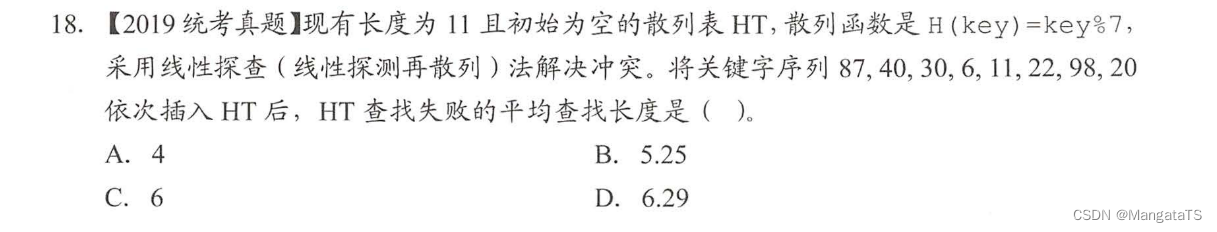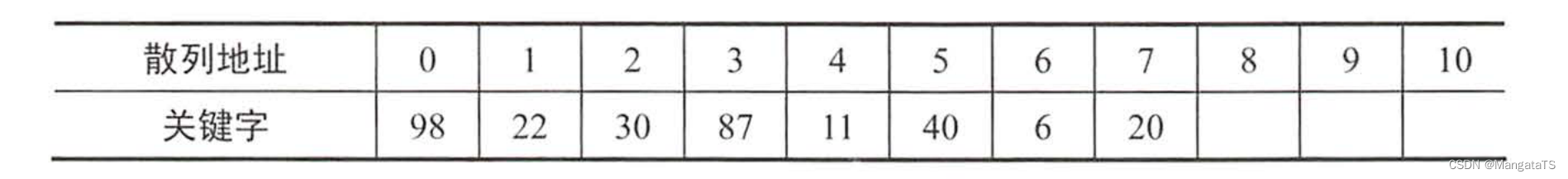# 哈希表|散列表¶

## 二、 哈希函数|散列函数¶

### 2.1 直接定址法¶

$H(key)=key \\ \text{或者是} \\ H(key) = a\times key + b$

### 2.2 保留余数法¶

$H(key) = key \% p$

### 2.5 折叠法¶

$187+848+780+52 = 1867$

## 三、冲突处理¶

### 3.1 开放定址法¶

$H_i = (H(key) + d_i) \% m$

#### 3.1.3 双散列法¶

$H_i = (H(key)+Hash_i(key)) \% m \\ \\ \text{或者是} \\ \\ H_i = (H(key) + i\times Hash_2(key)) \% m$

## 四、 性能分析¶

$ASL_{成功}=\sum_{i=1}^n\frac{(查找第 i 个元素比较的次数)}{n}$

$ASL_{失败}=\sum_{i=1}^n\frac{(散列表中关键字最大地址+1-插入的关键字的地址)}{n}$我们先将散列表画出来：假如我们查找的元素映射到地址 $$0$$ ，我们就需要从 $$0$$ 开始比对，直到找到地址 $$8$$ ，我们发现后面没关键字了，于是确定了我们查询的关键字不在散列表中，即查找失败，因为我们的散列函数只有 $$[0,7]$$ 这个区间，于是我们就得到了其失败平均查找长度：$$ASL_{失败} = \frac{9+8+7+6+5+4+3}{7} = 6$$